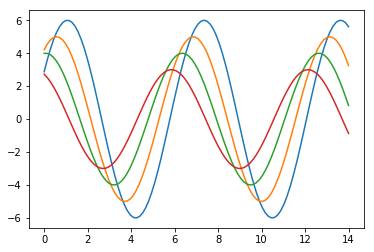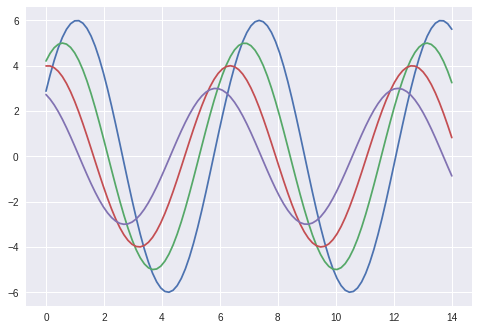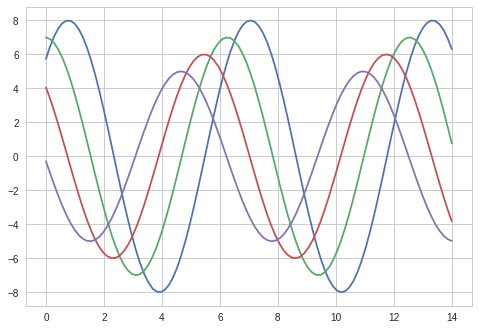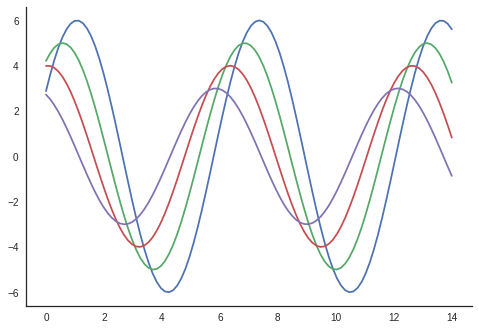# Seaborn Tutorial¶

Basic Introduction to Seaborn

Seaborn is an open-source, BSD-licensed Python library providing high-level API for visualizing the data using Python programming language.

This tutorial will take you through the basics and various functions of Seaborn. It is specifically useful for people working on data analysis. Once you have completed this tutorial, you will find yourself at a moderate level of expertise from where you can take yourself to higher levels of expertise.

Skills Required: You should have a basic understanding of computer programming terminologies. A basic understanding of Python and any of the programming languages is a plus. Seaborn library is built on top of Matplotlib. Having a basic idea of Matplotlib will help you understand this tutorial in a better way.

## Table of Content¶

#### Seaborn - Scaling Plot Elements¶

In the modern world of Data and Analytics, the most common and also one of the best ways to get insights is by visualizing the data. Data can be visualized by representing it as plots which are easy to understand, explore and grasp. Such data helps in drawing the attention of key elements.

To analyze a set of data using Python, we make use of Matplotlib, a widely implemented 2D plotting library. Likewise, Seaborn is a visualization library in Python. It is built on top of Matplotlib.

### Important Features of Seaborn¶

Seaborn is built on top of Python’s core visualization library Matplotlib. It is meant to serve as a compliment and not a replacement. However, Seaborn comes with some very important features. Let us see a few of them here. The features help in −

• Built-in themes for styling matplotlib graphics
• Visualizing univariate and bivariate data
• Fitting in and visualizing linear regression models
• Plotting statistical time-series data
• Seaborn works well with NumPy and Pandas data structures
• It comes with built-in themes for styling Matplotlib graphics

In most cases, you will still use Matplotlib for simple plotting. The knowledge of Matplotlib is recommended to tweak Seaborn’s default plots.

Data Visualization

Visualizing data is one step and further making the visualized data more pleasing is another step. Visualization plays a vital role in communicating quantitative insights to an audience to catch their attention.

Aesthetics means a set of principles concerned with the nature and appreciation of beauty, especially in art. Visualization is an art of representing data in an effective and easiest possible way.

Matplotlib library highly supports customization, but knowing what settings to tweak to achieve an attractive and anticipated plot is what one should be aware of to make use of it. Unlike Matplotlib, Seaborn comes packed with customized themes and a high-level interface for customizing and controlling the look of Matplotlib figures.

Basically, Seaborn splits the Matplotlib parameters into two groups−

• Plot styles
• Plot scale
In :
import numpy as np
from matplotlib import pyplot as plt
def sinplot(flip = 1):
x = np.linspace(0, 14, 100)
for i in range(1, 5):
plt.plot(x, np.sin(x + i * .5) * (7 - i) * flip)
sinplot()
plt.show()In :
import numpy as np
from matplotlib import pyplot as plt
def sinplot(flip = 1):
x = np.linspace(0, 14, 100)
for i in range(1, 5):
plt.plot(x, np.sin(x + i * .5) * (7 - i) * flip)
import seaborn as sb
sb.set()
sinplot()
plt.show()## Seaborn Figure Styles¶

The interface for manipulating the styles is set_style(). Using this function you can set the theme of the plot. As per the latest updated version, below are the five themes available.

• Darkgrid
• Whitegrid
• Dark
• White
• Ticks

Let us try applying a theme from the above-mentioned list. The default theme of the plot will be darkgrid which we have seen in the previous example.

In :
import numpy as np
from matplotlib import pyplot as plt
def sinplot(flip=1):
x = np.linspace(0, 14, 100)
for i in range(1, 5):
plt.plot(x, np.sin(x + i * .8) * (9 - i) * flip)
import seaborn as sb
sb.set_style("whitegrid")
sinplot()
plt.show()## Scaling Plot Elements¶

We also have control over the plot elements and can control the scale of the plot using the set_context() function. We have four preset templates for contexts, based on relative size, the contexts are named as follows

• Paper
• Notebook
• Talk
• Poster

By default, context is set to notebook; and was used in the plots above.

In :
import numpy as np
from matplotlib import pyplot as plt
def sinplot(flip = 1):
x = np.linspace(0, 14, 100)
for i in range(1, 5):
plt.plot(x, np.sin(x + i * .5) * (7 - i) * flip)
import seaborn as sb

sb.set_style("white")
sinplot()
sb.despine()
plt.show()Dolly Solanki